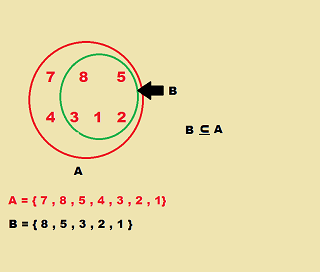# Sets in Math: Definition & Symbols - Quiz & Worksheet

Instructions:

question 1 of 3

### When talking about sets, what does the symbol 'U' mean?

Create Your Account To Take This Quiz

As a member, you'll also get unlimited access to over 84,000 lessons in math, English, science, history, and more. Plus, get practice tests, quizzes, and personalized coaching to help you succeed.

Try it risk-free for 30 days. Cancel anytime

### 1. What does this diagram show?### 2. If A = { 3 , 4 , 7 } and B = { 3 , 5 , 8 }, what is A U B?

Create your account to access this entire worksheet
Quizzes, practice exams & worksheets
Certificate of Completion
Create an account to get started

This multiple-choice quiz will challenge you to solve a handful of math problems involving sets. You'll also be asked to determine what particular set symbols signify.

## Quiz & Worksheet Goals

To pass the quiz, you'll need to:

• Understand what the 'U' symbol means
• Explain what an example diagram is showing
• Find A U B from two example sets
• Select a possible subset of an A set
• Tell what an upside down 'U' signifies

## Skills Practiced

You'll get practice using the following skills:

• Problem solving - apply your math skills to solve several problems involving sets
• Information recall - access knowledge you've gained regarding the 'U' and upside down 'U' symbols
• Knowledge application - use what you know about sets to determine what an example diagram shows

• Define sets and subsets
• See how sets can intersect
• Work through a sample problem using a set of books
Final ExamMath for Kids
Status: Not Started
Chapter ExamWorking with Data for Elementary School
Status: Not Started

Support